# High School Math : How to find the volume of a prism

## Example Questions

### Example Question #91 : Solid Geometry

Find the volume of the following triangular prism.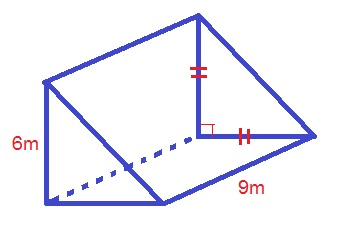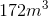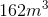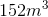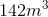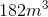Explanation:

The formula for the volume of a triangular prism is: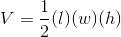Whereis the length of the triangle,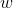is the width of the triangle, andis the height of the prism

Plugging in our values, we get: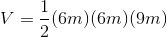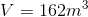### Example Question #92 : Solid Geometry

Find the volume of the following triangular prism.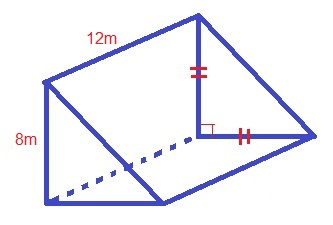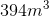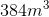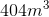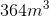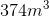Explanation:

The formula for the volume of a triangular prism is: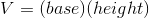Whereis the length of the base,is the width of the base, andis the height of the prism

Plugging in our values, we get: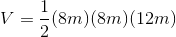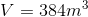### Example Question #93 : Solid Geometry

Find the volume of the following triangular prism: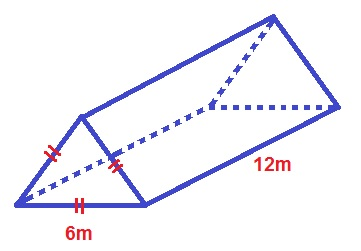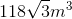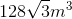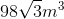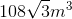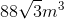Explanation:

The formula for the volume of an equilateral, triangular prism is: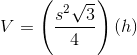Whereis the length of the triangle side andis the length of the height.

Plugging in our values, we get: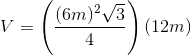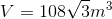### Example Question #94 : Solid Geometry

What is the volume?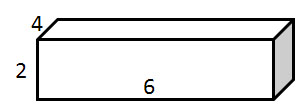Explanation:

The volume is calculated using the equation: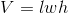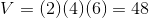### Example Question #1 : How To Find The Volume Of A Prism

A rectangular box has two sides with the following lengths:andIf it possesses a volume of, what is the area of its largest side?

49

28

16

12

21

28

Explanation:

The volume of a rectangular prism is found using the following formula:If we substitute our known values, then we can solve for the missing side.Divide both sides of the equation by 12.We now know that the missing length equals 7 centimeters.

This means that the box can have sides with the following dimensions: 3cm by 4cm; 7cm by 3cm; or 7cm by 4cm. The greatest area of one side belongs to the one that is 7cm by 4cm.